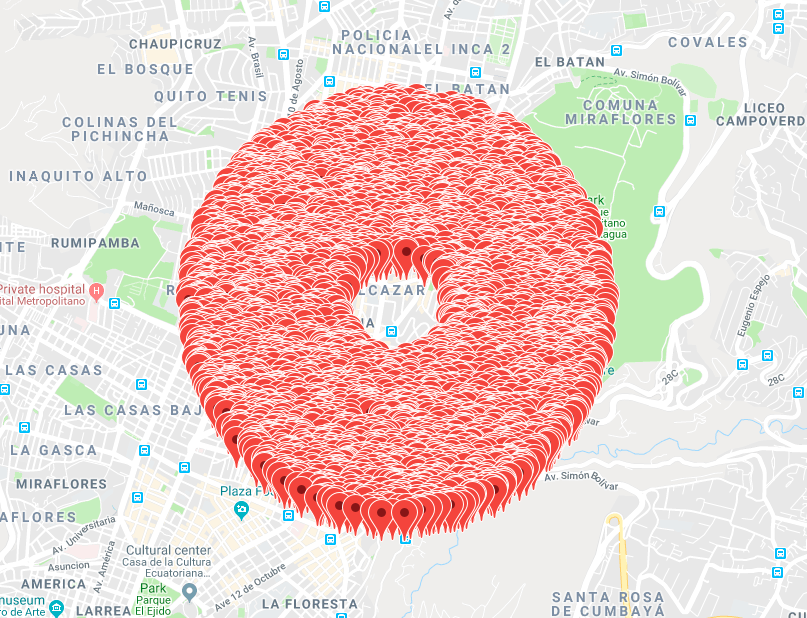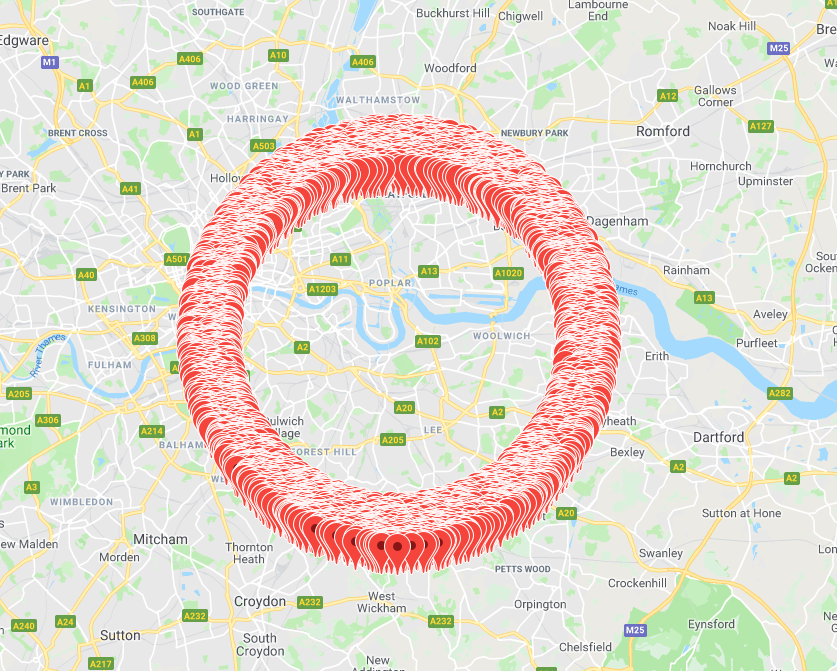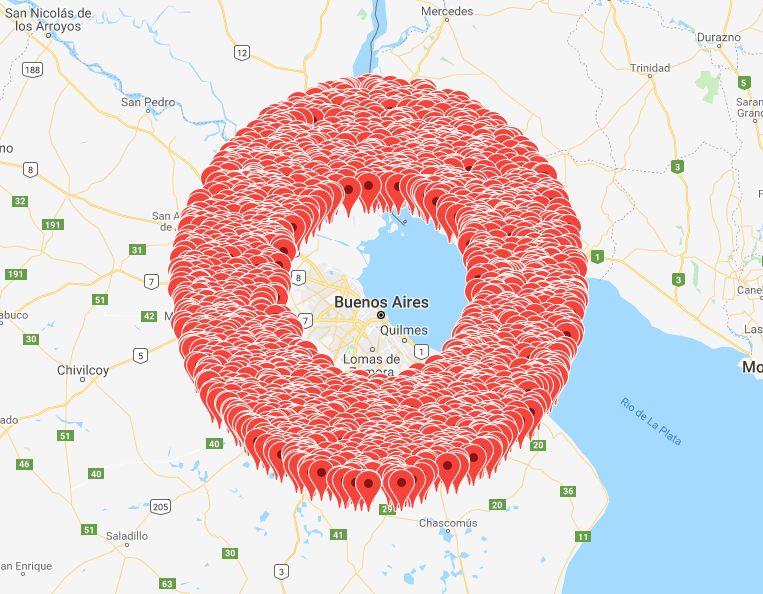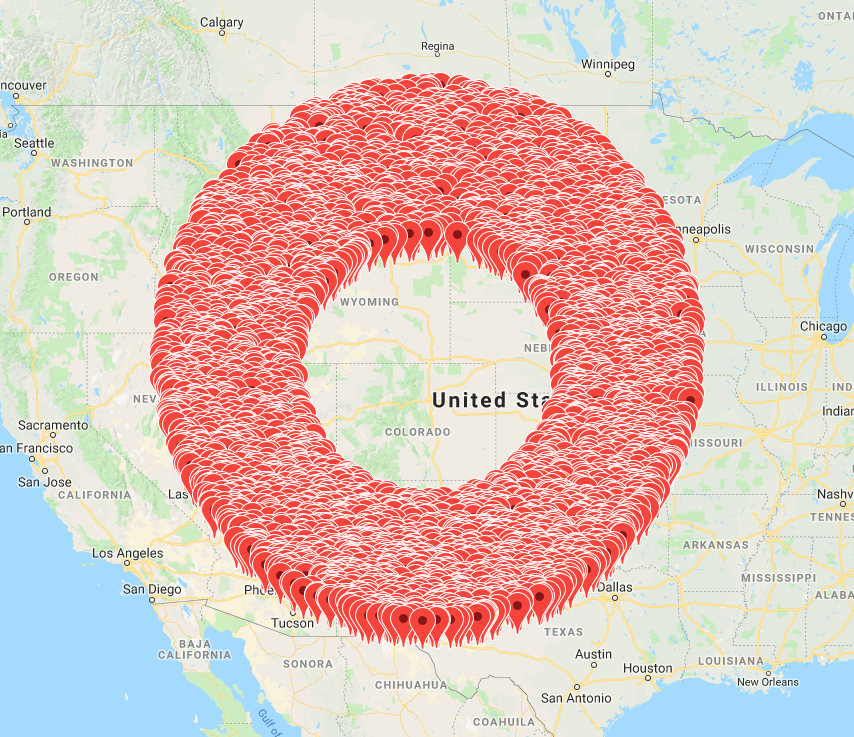### Introduction

On a previous project we needed to show markers on a map to indicate there was a property, but for privacy reasons we wanted to show the marker on the map close to the property but not at the exact location of the property. So we needed to generate random points within a fixed distance of the original point location. As you would expect, I did some Googling first but, to my surprise, the solutions I found didn’t produce something that looked like a circle when generating many points at exactly a given radius, so they were far from good in my opinion.

In conclusion, I had to create a better solution and that’s when I decided to apply…

### The Haversine Formula

The Haversine Formula is used to calculate the spherical distance $$d$$ on a sphere of radius $$R$$ between two points with latitude and longitude $$\left(\varphi_1, \lambda_1\right)$$ and $$\left(\varphi_2, \lambda_2\right)$$: $$hav \ \frac{d}{R} = hav \left(\varphi_2 - \varphi_1\right) + \cos \varphi_1 \cos \varphi_2 \ hav \left(\lambda_2 - \lambda_1\right)$$ Where $$hav \ \alpha = \frac{1 - \cos \alpha}{2}$$ And therefore \begin{align} - \cos \frac{d}{R} &= - \cos \left(\varphi_2 - \varphi_1\right) + \cos \varphi_1 \cos \varphi_2 \left(1 - \cos \left(\lambda_2 - \lambda_1 \right)\right) \\ &= - \sin \varphi_1 \sin \varphi_2 - \cos \varphi_1 \cos \varphi_2 \cos \left(\lambda_2 - \lambda_1 \right) \end{align} Finally $$d = R \arccos \left(\sin \varphi_1 \sin \varphi_2 + \cos \varphi_1 \cos \varphi_2 \cos \left(\lambda_2 - \lambda_1 \right)\right)$$

### Generating random points

Instead of using the Haversine formula to find the distance $$d$$ between two points $$\left(\varphi_1, \lambda_1\right)$$ and $$\left(\varphi_2, \lambda_2\right)$$, we're going to use it to find all points $$\left(\varphi_2, \lambda_2\right)$$ whose distance from $$\left(\varphi_1, \lambda_1\right)$$ is $$d$$.

Note $$0 \lt d \lt \pi R$$, $$-\frac{\pi}{2} \lt \varphi_i \le \frac{\pi}{2}$$ and $$- \pi \lt \lambda_i \le \pi$$. We're also going to define $$\Delta \varphi = \varphi_2 - \varphi_1$$ and $$\Delta \lambda = \lambda_2 - \lambda_1$$ and will be solving the equation for $$\Delta \varphi$$ and $$\Delta \lambda$$ instead.

So let's start with $$\cos \frac{d}{R} = \sin \varphi_1 \sin \varphi_2 + \cos \varphi_1 \cos \varphi_2 \cos \left(\lambda_2 - \lambda_1 \right)$$ We can isolate $$\Delta \lambda$$ $$\Delta \lambda = \pm \arccos \frac{\cos \frac{d}{R} - \sin \varphi_1 \sin \varphi_2}{\cos \varphi_1 \cos \varphi_2}$$ And arccos only takes values between -1 and 1, therefore: $$-1 \le \frac{\cos \frac{d}{R} - \sin \varphi_1 \sin \varphi_2}{\cos \varphi_1 \cos \varphi_2} \le 1$$ From the right hand side inequality \begin{align} \cos \frac {d}{R} \le \ &\cos \varphi_1 \cos \varphi_2 + \sin \varphi_1 \sin \varphi_2 =\\ & \cos \left(\varphi_2 - \varphi_1\right) = \cos \Delta \varphi \end{align} Therefore, given $$0 \lt d \lt \pi R$$ $$- \frac{d}{R} \le \Delta \varphi \le \frac {d}{R}$$ From the left hand side inequality \begin{align} \cos \frac {d}{R} \ge \ &- \cos \varphi_1 \cos \varphi_2 + \sin \varphi_1 \sin \varphi_2 =\\ & - \cos \left(\varphi_1 + \varphi_2\right) = - \cos \left(\Delta \varphi + 2 \varphi_1 \right) \end{align} Hence $$\cos \left(\Delta \varphi + 2 \varphi_1 \right) \ge - \cos \frac {d}{R} = \cos \left(\pi + \frac {d}{R}\right)$$ Therefore, given $$0 \lt d \lt \pi R$$ and $$-\frac{\pi}{2} \lt \varphi_i \le \frac{\pi}{2}$$ $$- \frac{d}{R} \le \Delta \varphi \le \frac {d}{R}$$ In conclusion, We can generate points $$\left(\varphi_2, \lambda_2\right)$$ whose distance from $$\left(\varphi_1, \lambda_1\right)$$ is $$d$$ with $$- \frac{d}{R} \le \Delta \varphi \le \frac {d}{R} \\ \Delta \lambda = \pm \arccos \left(\frac{\cos \frac{d}{R} - \cos \Delta \varphi}{\cos \varphi_1 \cos \left(\Delta \varphi + \varphi_1\right)} + 1\right) \\ \varphi_2 = \varphi_1 + \Delta \varphi, \ \lambda_2 = \lambda_1 + \Delta \lambda$$ Note 1 We won't get results for $$\Delta \lambda$$ above when $$\varphi_1 = \pm \frac{\varphi}{2}$$, that is for the poles.

Note 2 We're using spherical coordinates, but the Earth is not a perfect sphere, so this just an approximation.

### Ruby Implementation

The code below will generate a new point that’s more than 100 meters away but less than 200 meters away from the original point. Notice we convert the input from degrees to radians and the output from radians to degrees. Also, it would probably be a good idea to use the big decimal library to avoid floating point errors. And lastly, if this is for a web and you need map markers to be at the same spot across refreshes you will have to use a pseudo-random number generator.

Edit (18-02-2019): as pointed out in a discussion on Reddit, to generate points uniformly across each circle, \Delta \varphi needs to be d / R \ \cos x where x is a random number between 0 and \pi.

Edit (19-02-2019): Also from the same discussion on Reddit, to generate a uniform ditribution accross the radius, we need to generate points at distance \sqrt \left(x \left(d_max^2 - d_min^2\right) + d_min^2 \right) where x is a random number between 0 and 1.

lat = (point * Math::PI / 180).to_d
lon = (point * Math::PI / 180).to_d
max_distance = 200.to_d
min_distance = 100.to_d
distance = Math.sqrt(rand * (max_distance ** 2 - min_distance ** 2) + min_distance ** 2)

delta_lat = Math.cos(rand *  Math::PI) * distance / earth_radius
sign = rand(2) * 2 - 1
delta_lon = sign * Math.acos(
(Math.cos(lat) * Math.cos(delta_lat + lat))) + 1)

[(lat + delta_lat) * 180 / Math::PI, (lon + delta_lon) * 180 / Math::PI]


### ExamplesPoints between 100 meters and 200 meters away from the Eiffel Tower, Paris (France)Points between 1.5km and 2km away from Quito (Ecuador)Points between 8km and 10km away from the Greenwich Observatory, London (UK)Points between 50km and 100km away from Buenos Aires (Argentina)Points between 500km and 1000km away from Denver (Colorado)